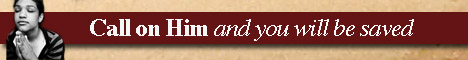Note:  Do not rely on this information. It is very old.

# Pascals Theorem

Pascal's Theorem was enunciated when its author was only a boy of sixteen, and first appeared in a short essay published in 1640. It states that if a hexagon be inscribed in a conic the intersections of opposite sides are three points in a line. This line is called the Pascal line. Any six points may be chosen, and they may be taken in any order to form a hexagon; hence, the hexagon may assume the most curious shape, quite different from the convex figure occurring in Euclidian geometry. In the adjoining figure abcdef is the hexagon; the opposite sides A B and d e meet at P, B C and E F meet at Q, while c D and F A meet at R. The points p Q R are seen to be collinear, p R being the Pascal line. Those same six points may be taken in many different orders, giving, in fact, as many as sixty different hexagons with a Pascal line for each. If five points abode are given they are sufficient to completely determine the conic which shall pass through them; hence, if any line be drawn through one of these points - E, for example - we can from Pascal's theorem find a point F on this line which shall also lie on the conic. Since this can be repeated any number of times, we can obtain any number of points on the conic and so be enabled to draw the curve.